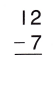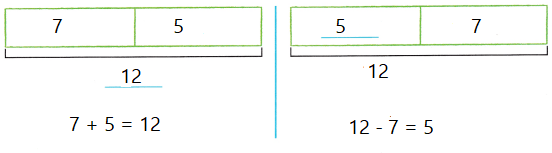Refer to our Texas Go Math Grade 2 Answer Key Pdf to score good marks in the exams. Test yourself by practicing the problems from Texas Go Math Grade 2 Lesson 5.3 Answer Key Connect Subtraction to Addition.

Explore

Complete the model to show the problem.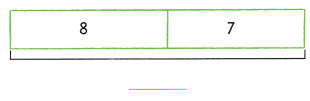___________ soccer balls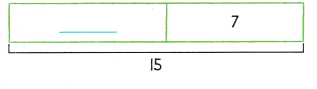___________ soccer ballsExplanation:
Addition and Subtractions are inverse operations,
which are shown in the above equations.

FOR THE TEACHER • Read the following problems. Have children complete the model for each. The soccer team has 8 red balls and 7 yellow balls. How many soccer balls does the team have? The soccer team has 15 balls inside the locker room. The children took the 7 yellow balls outside. How many soccer balls were inside?
Total soccer balls are 15 balls.
Number of soccer balls inside are 8 balls.
Explanation:
Addition and Subtractions are inverse operations,
which are shown in the below equations.Math Talk
Mathematical Processes

Explain how the models for the problems are alike and how they are different.
The models for the problems are alike as we use addition facts to remember differences. Related facts have the same whole and parts the difference.

Model and Draw

You can use addition facts to remember differences. Related facts have the same whole and parts.
Think of the addends in an addition fact to find the difference for a related subtraction fact.
Write the missing numbers.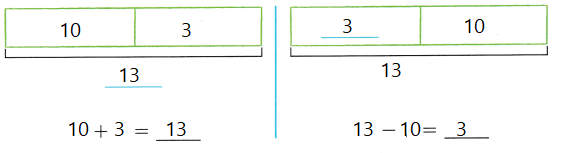Explanation:
The missing numbers are 13 and 6
Both the above equations are inverse to each other.

Share and Show

Write the difference.

Question 1.
5 – 2 = _____________Explanation:
Addition and Subtractions are inverse operations,
which are shown in the above equations.
Question 2.
8 – 4 = _____________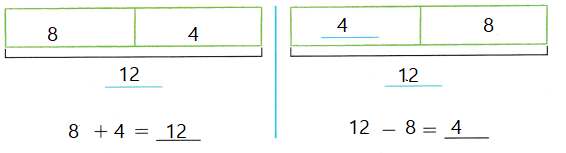Explanation:
Addition and Subtractions are inverse operations,
which are shown in the above equations.
The addends in an addition fact helps to find the difference for a related subtraction fact.

Question 3.
_____________ = 7 – 6Explanation:
The addends in an addition fact helps to find the difference for a related subtraction fact.
Addition and Subtractions are inverse operations,
which are shown in the above equations.

Question 4.
10 – 3 = _____________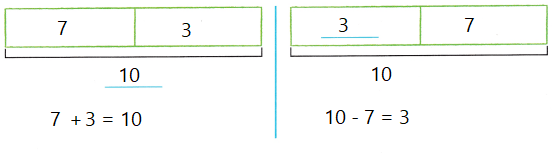Explanation:
Addition and Subtractions are inverse operations,
which are shown in the above equations.
The addends in an addition fact helps to find the difference for a related subtraction fact.

Question 5.
_____________ = 9 – 6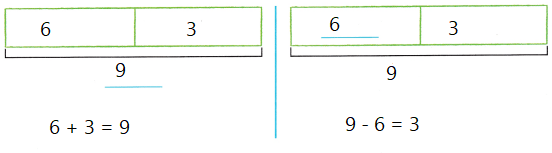Explanation:
The addends in an addition fact helps to find the difference for a related subtraction fact.
Addition and Subtractions are inverse operations,
which are shown in the above equations.

Question 6.
11 – 4 = _____________Explanation:
The addends in an addition fact helps to find the difference for a related subtraction fact.
Addition and Subtractions are inverse operations,
which are shown in the above equations.

Question 7.
9 – 7 = _____________Explanation:
Addition and Subtractions are inverse operations,
which are shown in the above equations.
The addends in an addition fact helps to find the difference for a related subtraction fact.

Question 8.
13 – 4 = _____________Explanation:
The addends in an addition fact helps to find the difference for a related subtraction fact.
Addition and Subtractions are inverse operations,
which are shown in the above equations.

Question 9.
_____________ = 15 – 7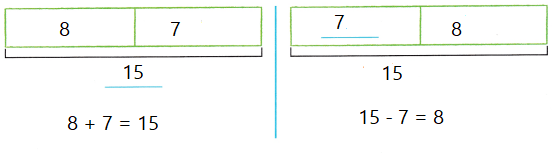Explanation:
The addends in an addition fact helps to find the difference for a related subtraction fact.
Addition and Subtractions are inverse operations,
which are shown in the above equations.

Question 10.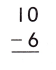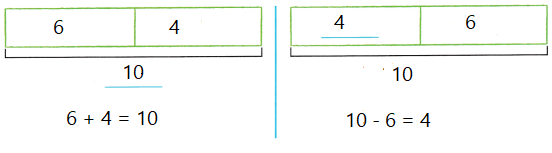Explanation:
Addition and Subtractions are inverse operations,
which are shown in the above equations.
The addends in an addition fact helps to find the difference for a related subtraction fact.

Question 11.Explanation:
Addition and Subtractions are inverse operations,
which are shown in the above equations.
The addends in an addition fact helps to find the difference for a related subtraction fact.

Question 12.